Next: MCMC Up: Appendix Previous: Appendix

## Gamma Distribution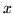has a two-parameter gamma distribution, denoted by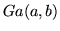, with parametersand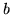, if its density is given by: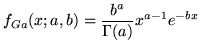(26)

where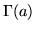is the Gamma function. Note, that a gamma distribution hasand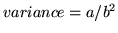. The mode of the Gamma distribution is given by: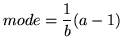(27)